# Relations and functions Worksheets with solutions

## Fill in the blank

(a) The Cartesian product $P \times P$ has 9 elements among which are found (-2, 0) and (0, 2). the set P is _____ and the remaining elements of P × P__________
(b) The function f(x) is defined as $x^2$ . The value of $\frac {f(2) -f(1)}{2-1}$ ______
(c) The function p(x)=x+1 and q(x)=2x-1.The value (f/g)x is ______
(d) The Function g(x)=6x2+3x-2 The value of g(-1) is ________

## True or False statement

(1) The relation defined as {(2, 1), (5, 1), (8, 1), (11, 1), (14, 1), (17, 1)} is a function
(2) The relation defined as {(2, 1), (4, 2), (6, 3), (8, 4), (10, 5), (12, 6), (14, 7)} is not a function
(3) The relation defined as {(1, 3), (1, 5), (2, 5)} is a function
(4) The below graph is not a function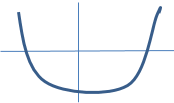(5) A function are relations but all relations are not functions
(6) A function is defined as
$f(x) =- \sqrt {-2x+5}$
The domain is x≤ 5/2 and Range is f(x) ≤ 0
(7) P= {1,2,3} Q={e,f}. The total number of relation from P × Q is 64
(8) The below graph is a function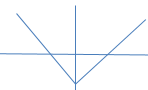(9) The below graph is not a function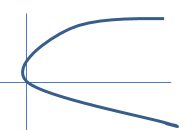(10) The ordered pair {(x,y)|y < 3x+1} is a function
(11) The ordered pair {(x,y)|y=x2} is a relation but not function
(12) The ordered pair {(x,y)| x=3 and y is real number} is a relation and function

## Subjective Questions

Find the domain and range of the following real function:
(1) $y=x^2$
(2) $y=-|x|$
(3) $y=3x-7$
(4) $y=-x^4 + 3$
(5) $y= \sqrt {2-x}$
(6) $y =\frac {1}{ \sqrt {11-x}}$

If A= {1,2,3, 5, 7, 9, 11}, B = {7, 9, 11, 13}, C = {11, 13, 15} D = { 17,19,21,23} and E={1,-1} find
(i) What is the number of element in $A \times B$
(ii) How many number of relations can be found from $A \times C$
(iii) The mapping defined as {(1,11),{1,13},{2,7},{5,11} is a function from A × B,State True or False
(iv) The mapping defined as {(11,17),(13,19),(15,21),(15,23)} is a Relation from C × D, State True or False
(v) Find the value of B × C and C × B
(vi) Find the value of E × E × E
(vii) Verify that A × (B ∩ C) = (A × B) ∩ (A × C)
(viii) A X B is a subset of A X C. State True or false
(ix) (A X B) ∩ (B ∪ D)

## Mulitiple Choice questions

1. Given the relation R = {(6,4), (8,-1), (x,7), (-3,-6)}. Which of the following values for x will make relation R a function?
(a) 8
(b) 6
(c) -3
(d) 1

2. Let n (A) = m, and n (B) = n. Then the total number of non-empty relations that can be defined from A to B is
(a) $m^n$
(b) mn- 1
(c) $2^{mn} -1$
(d) $n^m -1$

3. The domain and range of real function f defined by f (x) = $\sqrt {x −1}$ is given by
(A) Domain = (1, ∞), Range = (0, ∞)
(B) Domain = [1, ∞), Range = (0, ∞)
(C) Domain = [1, ∞), Range = [0, ∞)
(D) Domain = [1, ∞), Range = [0, ∞)

4. If $f (x) = x^3 - \frac {1}{x^3}$ then $f (x) + f(\frac {1}{x})$
(a) 0

(b) $2x^3$
(c) 1
(d) $\frac {2}{x^3}$

## Subjective question

1. What is the domain and range in the relation shown in below mapping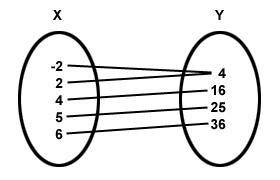2. Please tell if the below mapping is function or not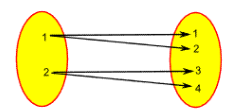There are two functions defined as below
Let P={(0,5),(1,4),(2,3),(3,2),(4,1),(5,0)}
Q={(1,1),(2,4),(3,9),(4,16),(5,25),(6,36)}
1.What is the domain and range of P
2.What is the domain and range of Q
3. What is the domain of function (Q-P)
4. List the ordered pair of (Q-P) in set notation
5. What is the domain of Q/P
6. List the ordered pair of (Q/P) in set notation